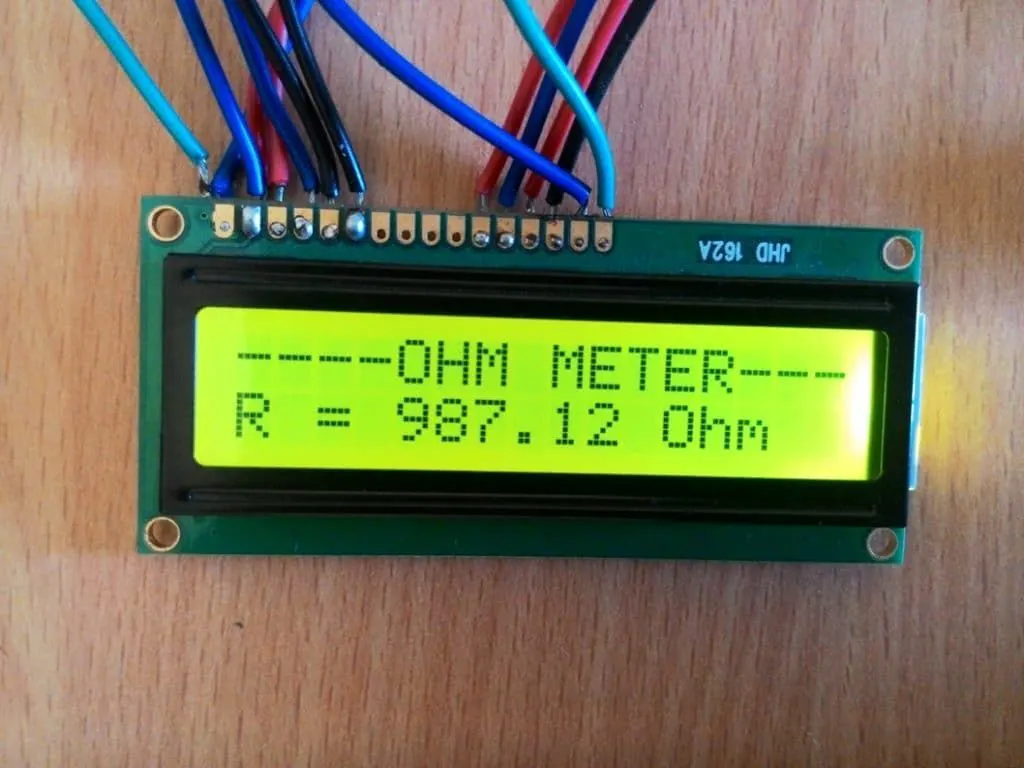Project tutorial# How to make an Arduino Ohm Meter © LGPL

I am going to present an easy way to know the values of resistors without reading the color bands on them. You'll never need to guessing!

• 22,511 views
• 18 respects

## Apps and online services

### WIRING THE OHM METER

The circuit is very simple. All you need is an Arduino, the resistor you want to measure, and another resistor with a known value. We’ll set up a voltage divider with the known and unknown resistors, and measure the voltage between them with the Arduino. Then we’ll run a program that will calculate the resistance from Ohm’s Law.

First, wire up the circuit like this:

Enter the value of your known resistor (in Ohms) on line 5 of the code above. In my case, I’m using a known resistor with a value of 1K Ohms (1000 Ohms). Therefore, my line 5 should look like this: float R1 = 1000;.

The program sets up analog pin A0 to read the voltage between the known resistor and the unknown resistor. You can use any other analog pin though, just change the pin number in line 1, and wire the circuit accordingly.

When you open up the serial monitor you’ll see the resistance values printed once per second. There will be two values, R2 and Vout.

• R2: is the resistance of your unknown resistor in Ohms.
• Vout: is the voltage drop across your unknown resistor.

### OUTPUT THE READINGS TO AN LCD

One reader commented that they would like to display the resistance measurements on an LCD. This is easy to do. First you’ll want to read our tutorial on setting up an LCD display on the Arduino. That will show you how to connect everything.

### HOW ACCURATE IS IT?

These are the readings I got with a 200 Ohm “unknown” resistor:

The values are pretty accurate, that’s only an error of 1.6%.

However, these are the readings I got when I measured a 220K Ohm “unknown” resistor:

The error here is greater than 100%.

That’s because I was still using a 1K Ohm known resistor. The accuracy of the Ohm meter will be poor if the value of the known resistor is much smaller or larger than the resistance of the unknown resistor.

The problem is easily fixed by using a known resistor that’s closer in value to the unknown resistor. Once I replaced the 1K Ohm known resistor with a 100K Ohm resistor, the accuracy of the measurements improved greatly.

Don’t forget to change line 5 in the code above (float R1 = 1000) to the value of your new known resistor.

These are the values I got with the same 220K Ohm “unknown” resistor and a 100K Ohm known resistor:

Finally, all the known resistors have an error 1.6-200%.But we can easily measure resistors without having a multimeter.

## Code

##### CODE FOR SERIAL MONITOR OUTPUT OF MEASURMENT RESISTOR:C/C++
Now, enter this code into the Arduino IDE and upload it to your board:
```int analogPin= 0;
int raw= 0;
int Vin= 5;
float Vout= 0;
float R1= 1000;
float R2= 0;
float buffer= 0;

void setup()
{
Serial.begin(9600);
}

void loop()
{
if(raw)
{
buffer= raw * Vin;
Vout= (buffer)/1024.0;
buffer= (Vin/Vout) -1;
R2= R1 * buffer;
Serial.print("Vout: ");
Serial.println(Vout);
Serial.print("R2: ");
Serial.println(R2);
delay(1000);
}
}
```
##### CODE FOR LCD OUTPUT OF MEASURING RESISTOR :C/C++
One reader commented that they would like to display the resistance measurements on an LCD. This is easy to do. First you’ll want to read our tutorial on setting up an LCD display on the Arduino. That will show you how to connect everything. After you’ve got the LCD set up, connect the Ohm meter as shown above, and upload this program to the Arduino:
```#include <LiquidCrystal.h>
LiquidCrystal lcd(12, 11, 5, 4, 3, 2);

int analogPin= 0;
int raw= 0;
int Vin= 5;
float Vout= 0;
float R1= 1000;
float R2= 0;
float buffer= 0;

void setup()
{
lcd.begin(16, 2);
}

void loop()
{
if(raw)
{
buffer= raw * Vin;
Vout= (buffer)/1024.0;
buffer= (Vin/Vout) -1;
R2= R1 * buffer;

lcd.setCursor(0, 0);
lcd.print("Vout: ");
lcd.print(Vout);

lcd.setCursor(0, 1);
lcd.print("R2: ");
lcd.print(R2);
delay(1000);
}
}
```

#### Ohm meter with Arduino Uno

Project tutorial by xristos_xatz

• 7,013 views
• 13 respects

#### Arduino Ohm Meter

Project showcase by lucian_vdo

• 12,296 views
• 19 respects

#### Stereo NeoPixel Ring VU Meter

Project showcase by ericBcreator

• 122,039 views
• 214 respects

#### Arduino DIY Light Meter With BH1750 Sensor

Project tutorial by Nick Koumaris

• 14,799 views
• 7 respects

#### Light Meter To Measure Plant Growth Conditions

Project tutorial by Jennifer Deegan

• 5,936 views